Get inspired by the success stories of our students in IIT JAM MS, ISI  MStat, CMI MSc DS.  Learn More

# Tracing the Trace | ISI MStat 2016 PSB Problem 3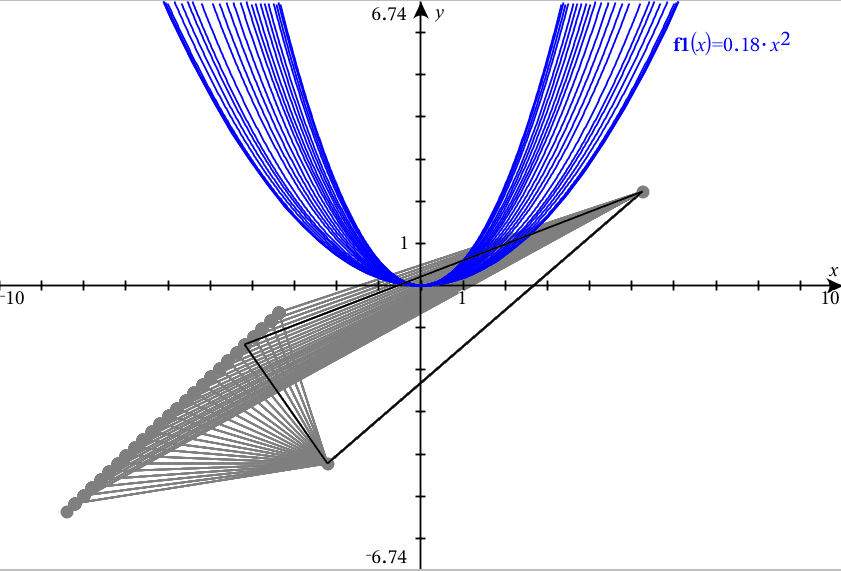This ISI MStat 2016 problem is an application of the ideas of tracing the trace and Eigen values of a matrix and using a cute sum of squares identity.

## Problem- Tracing the Trace

Suppose A is an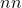real symmetric matrix such that. Show that all the eigenvalues of A are equal to 1.

This problem is from ISI MStat 2016 PSB ( Problem #3)

### Prerequisites

• Trace of a Matrix
• Eigen values of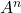w.r.t to the eigen values of.
• Sum of Squares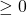.

## Solution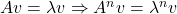.

Since, A is a real symmetric matrix, then all the eigen values of the matrix A are real say {}.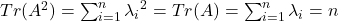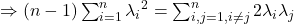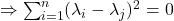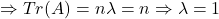.

This ISI MStat 2016 problem is an application of the ideas of tracing the trace and Eigen values of a matrix and using a cute sum of squares identity.

## Problem- Tracing the Trace

Suppose A is anreal symmetric matrix such that. Show that all the eigenvalues of A are equal to 1.

This problem is from ISI MStat 2016 PSB ( Problem #3)

### Prerequisites

• Trace of a Matrix
• Eigen values ofw.r.t to the eigen values of.
• Sum of Squares.

## Solution.

Since, A is a real symmetric matrix, then all the eigen values of the matrix A are real say {}..

This site uses Akismet to reduce spam. Learn how your comment data is processed.

### Knowledge Partner